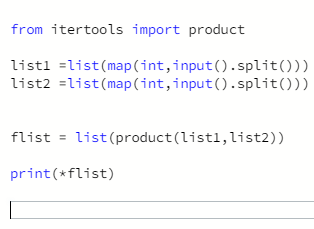# Hackerrank – Python – itertools.product() – Solution

Publish Date - March 19th, 2023

|

A pretty difficult question in my mind, and I was sent Googling for all different approaches for Python. I think the biggest issue is not having a strong understanding of iterools library and the product() function. Geekforgeeks, has a great walkthrough talkthrough on how this library works and it saved me! I can see this type of library being really good for all sorts of python applications (data science, ML) because you can import the functions to do the iterations for you. So instead of writing a normal loop or list comprehension, you can add a (*) on whatever you want to iterate on. Pretty cool if you’re looking to write clean code.

## The problem

itertools.product()

This tool computes the cartesian product of input iterables.
It is equivalent to nested for-loops.
For example, `product(A, B)` returns the same as `((x,y) for x in A for y in B)`.

Sample Code

``````>>> from itertools import product
>>>
>>> print list(product([1,2,3],repeat = 2))
[(1, 1), (1, 2), (1, 3), (2, 1), (2, 2), (2, 3), (3, 1), (3, 2), (3, 3)]
>>>
>>> print list(product([1,2,3],[3,4]))
[(1, 3), (1, 4), (2, 3), (2, 4), (3, 3), (3, 4)]
>>>
>>> A = [[1,2,3],[3,4,5]]
>>> print list(product(*A))
[(1, 3), (1, 4), (1, 5), (2, 3), (2, 4), (2, 5), (3, 3), (3, 4), (3, 5)]
>>>
>>> B = [[1,2,3],[3,4,5],[7,8]]
>>> print list(product(*B))
[(1, 3, 7), (1, 3, 8), (1, 4, 7), (1, 4, 8), (1, 5, 7), (1, 5, 8), (2, 3, 7), (2, 3, 8), (2, 4, 7), (2, 4, 8), (2, 5, 7), (2, 5, 8), (3, 3, 7), (3, 3, 8), (3, 4, 7), (3, 4, 8), (3, 5, 7), (3, 5, 8)]
``````

You are given a two lists  and . Your task is to compute their cartesian product X.

Example

``````A = [1, 2]
B = [3, 4]

AxB = [(1, 3), (1, 4), (2, 3), (2, 4)]
``````

Note:  and  are sorted lists, and the cartesian product’s tuples should be output in sorted order.

Input Format

The first line contains the space separated elements of list .
The second line contains the space separated elements of list .

Both lists have no duplicate integer elements.

Output Format

Output the space separated tuples of the cartesian product.

Sample Input

`````` 1 2
3 4
``````

Sample Output

`` (1, 3) (1, 4) (2, 3) (2, 4)``

## The solution

``````from itertools import product

list1 =list(map(int,input().split()))
list2 =list(map(int,input().split()))

flist = list(product(list1,list2))

print(*flist)
``````

Extremely simple code to start (with obviously the import from itertools).

You essentially have 2 inputs which are lists that have 2 variables each which you want as individual integers and broken up (since you’re starting w/ tuples). You want two lists in the end, so you’ll need to use the map function to be able to iterate further in the problem.

With the inputs taken care of, all you need to do is “iterate” with the product() function on the two lists and change that iteration back into two lists.

That being said, I didn’t understand the purpose of the (*), which is why I print it out at the end.